Units 11-12a Isle of Man Freeport, Ballasalla

# TN2020-05: Light as a wave

Light is a type of radiation consisting of vibrating electric and magnetic fields moving through space, much like how a sound wave is just a vibration travelling through the air. The electric and magnetic fields oscillate perpendicular to each other and to the direction of motion as depicted in figure 1.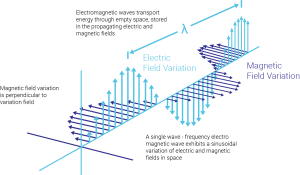Figure 1: A propagating electromagnetic wave. The magnetic and electric fields oscillate at right angles to each other and the direction of propagation.

Owing to its wave-like behaviour, light can be characterised by a number known as it’s wavelength which is just the physical length of a single cycle of oscillation. The different colours we perceive correspond to different wavelengths of light, however visible light only makes up a small part of what is known as the electromagnetic spectrum which is typically divided into gamma rays, x-rays, UV, visible light, infrared, microwaves and radiowaves. White light is simply all the different wavelengths of visible light travelling together. A light wave consisting of a single wavelength is known as “Monochromatic”.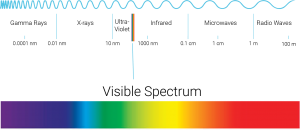Figure 2: The electromagnetic spectrum

A property of waves critical to optics is the phenomena known as “interference”. When two or more waves exist in the same area of space, they will combine with each other and produce a wave with modified properties. A simple example is two waves of the same wavelength and amplitude interfering constructively and destructively. When the waves are in phase, their peaks and troughs match each other and produce a wave with twice the amplitude. This is known as constructive interference. If the waves are 180deg out of phase (half a wavelength) then the peaks and troughs cancel. This is destructive interference.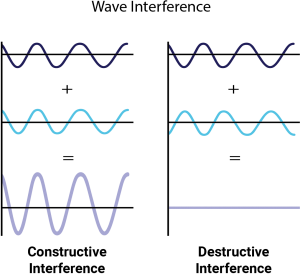Figure 3: Constructive and destructive interference. The in phase waves reinforce each other whereas the out of phase waves cancel out.

For many waves of different wavelengths, amplitudes and phases the interference becomes very complicated and the resultant wave may look quite messy. Virtually all precision optics from AR coated lens to Etalons to ultrafast mirrors exploit interference phenomena to achieve the desired functionality. Interference also plays an extremely important in the aptly named field of “interferometry” where interference effects are utilised to measure the flatness or shape of optics to an extreme level of precision.

Another important trait of waves is the phenomena referred to as diffraction. Diffraction broadly refers to the behaviour of light incident upon slits or obstructions. A helpful idea is Huygen’s principle which asserts that every point of a wave-front can be considered a source of secondary “wavelet’s” whose interference gives the resultant wave. When light passes through an aperture the interference of the wavelets causes diffraction. This is illustrated in figure 4 below.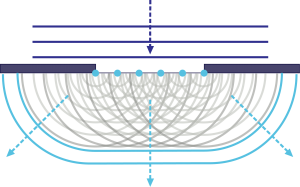Figure 4: Huygen’s principle in action. Wavelets on the wave-front passing through the aperture interfere to produce a more circular wave-front, the wave is thus diffracted as it passes through the slit.

Diffraction through a circular aperture causes the appearance of what is known as an “Airy Disk”, a bright spot surrounded by faint rings. The size of an airy disk for a given aperture is an important consideration in optical systems as it determines the smallest point to which light can be focused. A famous example illustrating both diffraction and interference principles is the double slit experiment depicted in figure 5.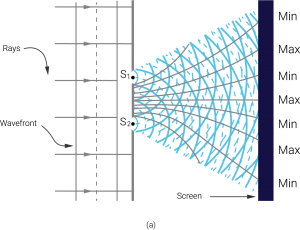Figure 5: The double slit experiment. A wave-front arrives at two slits and diffracts through. The diffraction causes two circular wave fronts to emit from the slits, these interfere to produce the famous interference pattern.

Handbook of Optical Systems Vol. 1, B. Dörband, H. Müller, Wiley-VCH Verlag GmbH & Co. KGaA, Germany,

Weinheim (2005) ISBN 978-3-527-40377-6

Handbook of Optical Systems Vol. 2, B. Dörband, H. Müller, Wiley-VCH Verlag GmbH & Co. KGaA, Germany,

Weinheim (2012) ISBN 978-3-527-40378-3